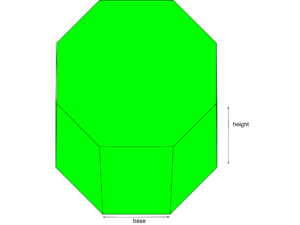# Program to find Surface Area and Volume of Octagonal Prism

Given the base length, height, and distance of Octagonal Prism we have to find the Surface Area and Volume of the octagonal prism for the given parameters. The octagonal prism is a solid three-dimensional object having a total of 10 faces, 24 edges, and 16 vertices. The two faces at either end are octagons and the rest of the faces are rectangular.where a is the base length, h is height and d is the distance of octagonal prism.

Area = 2 * base * distance
Surface area = (2 * Area) + ( 8 * base * height )
Volume = ( Area * height )

Examples:

Input: h = 2, a = 4, d = 3
Output:
Surface Area : 112
Volume : 48
Input: h = 1, a = 6, d = 2
Output:
Surface Area : 96
Volume : 24

## C++

 `// C++ program to find the ` `// Surface area and volume ` `// of octagonal prism ` `#include ` `using` `namespace` `std;`   `// Function to find the ` `// Volume of octagonal prism ` `void` `find_volume(``float` `area, ``float` `h)` `{ ` `    ``// Formula to calculate ` `    ``// volume = (area * h) ` `    ``float` `Volume = (area * h);` `    `  `    ``// Display volume ` `    ``cout << ``"Volume: "` `         ``<< Volume << endl;` `}` `    `  `// Function to find the ` `// surface area of octagonal prism ` `void` `find_Surface_area(``float` `area,` `                       ``float` `a, ``float` `h)` `{` `        `  `    ``// Formula to calculate Surface area ` `    ``float` `Surface_area = (2 * area) +` `                         ``(8 * a * h);` `    `  `    ``cout << ``"Surface area: "` `         ``<< Surface_area << endl;` `}`   `// Driver Code ` `int` `main() ` `{` `    ``float` `h = 1;` `    ``float` `a = 6;` `    ``float` `d = 2;` `    ``float` `area = 2 * a * d;` `    `  `    ``find_Surface_area(area, a, h);` `    `  `    ``find_volume(area, h);` `    `  `    ``return` `0;` `}`   `// This code is contributed by AnkitRai01`

## Java

 `// Java program to find the ` `// Surface area and volume ` `// of octagonal prism ` `public` `class` `GFG {`   `    ``// Function to find the ` `    ``// Volume of octagonal prism ` `    ``static` `void` `find_volume(``double` `area,``double` `h){ ` `        `  `        ``// Formula to calculate ` `        ``// volume = (area * h) ` `        ``double` `Volume = (area * h) ;` `        `  `        ``// Display volume ` `        ``System.out.println(``"Volume: "` `+ Volume);` `    ``}` `    `  `    ``// Function to find the ` `    ``// surface area of octagonal prism ` `    ``static` `void` `find_Surface_area(``double` `area, ``double` `a, ``double` `h){` `        `  `        ``// Formula to calculate Surface area ` `        ``double` `Surface_area = (``2` `* area)+(``8` `* a * h) ;` `        `  `        ``System.out.println(``"Surface area: "` `+ Surface_area) ;` `    ``}`   `    ``// Driver Code ` `    ``public` `static` `void` `main (String[] args) ` `    ``{` `        ``double` `h = ``1` `;` `        ``double` `a = ``6` `;` `        ``double` `d = ``2` `;` `        ``double` `area = ``2` `* a * d ;` `        `  `        `  `        ``find_Surface_area(area, a, h) ;` `        `  `        ``find_volume(area, h) ;` `    `  `    ``}` `}`   `// This code is contributed by AnkitRai01`

## Python3

 `# Python3 program to find the ` `# Surface area and volume ` `# of octagonal prism` `import` `math` `    `    `# Function to find the` `# Volume of octagonal prism` `def` `find_volume(area, h):` `    `  `    ``# Formula to calculate` `    ``# volume = (area * h)` `    ``Volume ``=` `(area ``*` `h)` `    `  `    ``# Display volume` `    ``print``(``"Volume: "``, end ``=``" "``)` `    ``print``(Volume)`   `# Function to find the` `# surface area of octagonal prism` `def` `find_Surface_area(area, a, h):` `    `  `    ``# Formula to calculate Surface area ` `    ``Surface_area ``=` `(``2` `*` `area)``+``(``8` `*` `a ``*` `h)` `    `  `    ``print``(``"Surface area: "``, end ``=``" "``) ` `    ``print``(Surface_area)` `    `  `# Driver Code` `h ``=` `1` `a ``=` `6` `d ``=` `2` `area ``=` `2` `*` `a ``*` `d`     `find_Surface_area(area, a, h)`   `find_volume(area, h)`

## C#

 `// C# program to find the surface area ` `// and volume of octagonal prism ` `using` `System;`   `class` `GFG { `   `// Function to find the volume ` `// of octagonal prism ` `static` `void` `find_volume(``double` `area, ``double` `h)` `{ ` `        `  `    ``// Formula to calculate ` `    ``// volume = (area * h) ` `    ``double` `Volume = (area * h); ` `        `  `    ``// Display volume ` `    ``Console.WriteLine(``"Volume: "` `+ Volume); ` `} ` `    `  `// Function to find the surface  ` `// area of octagonal prism ` `static` `void` `find_Surface_area(``double` `area, ``double` `a,` `                                           ``double` `h)` `{ ` `        `  `    ``// Formula to calculate surface area ` `    ``double` `Surface_area = (2 * area) + (8 * a * h); ` `    `  `    ``Console.WriteLine(``"Surface area: "` `+ Surface_area); ` `} `   `// Driver Code ` `public` `static` `void` `Main (``string``[] args) ` `{ ` `    ``double` `h = 1; ` `    ``double` `a = 6; ` `    ``double` `d = 2; ` `    ``double` `area = 2 * a * d; ` `    `  `    ``find_Surface_area(area, a, h); ` `    ``find_volume(area, h); ` `} ` `} `   `// This code is contributed by AnkitRai01 `

## Javascript

 ``

Output:

```Surface area:  96
Volume:  24```

Time complexity: O(1) because performing constant operations

Auxiliary Space: O(1)

Feeling lost in the world of random DSA topics, wasting time without progress? It's time for a change! Join our DSA course, where we'll guide you on an exciting journey to master DSA efficiently and on schedule.
Ready to dive in? Explore our Free Demo Content and join our DSA course, trusted by over 100,000 geeks!